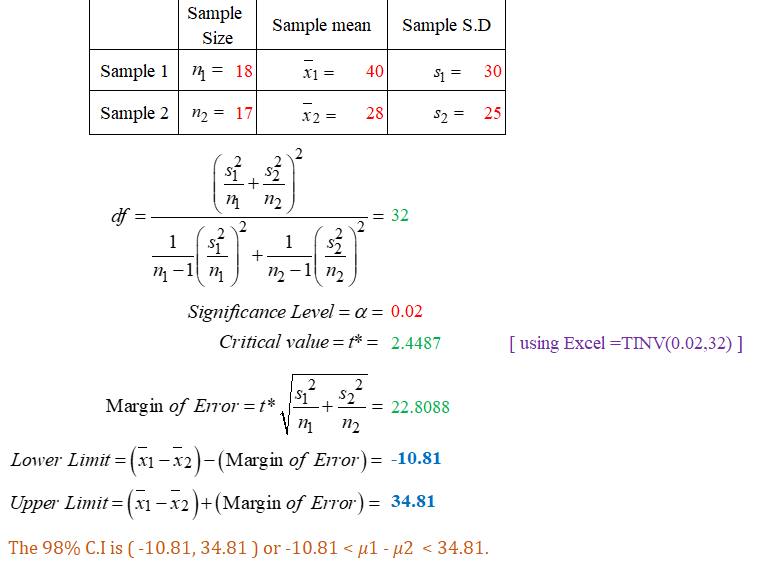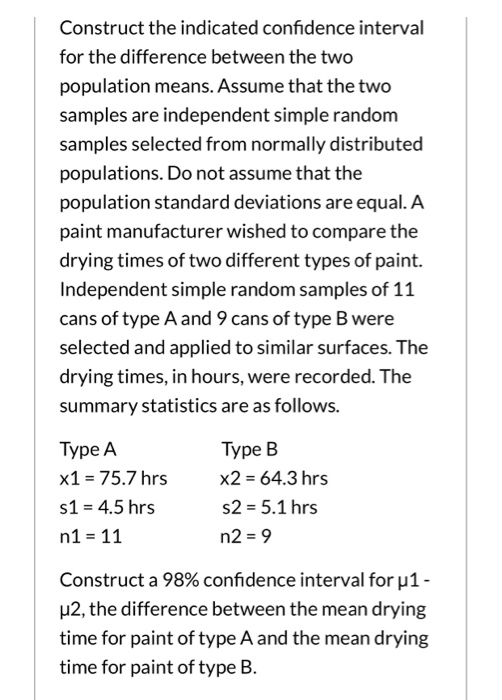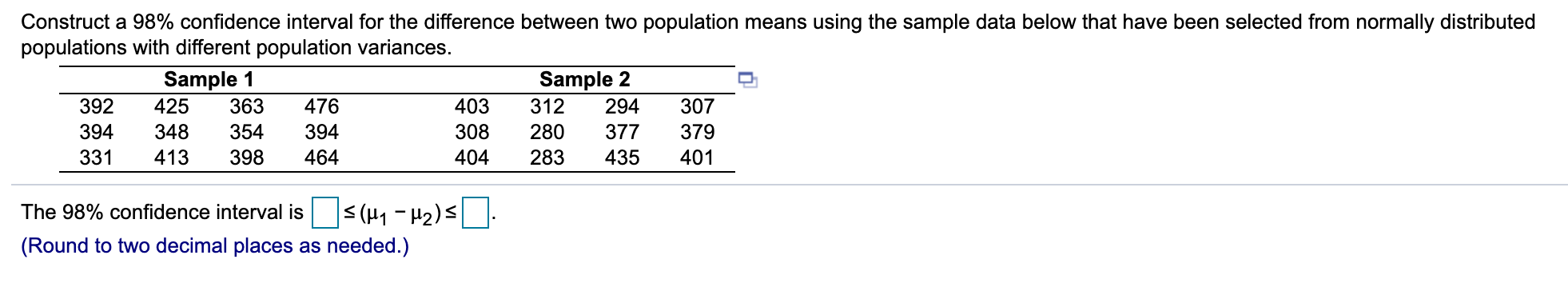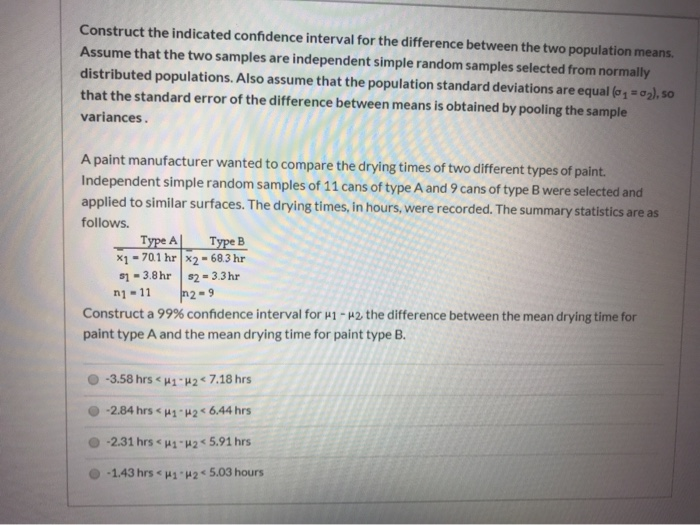Question

# Find the 98% confidence interval for the difference between two means based on this information about...

Find the 98% confidence interval for the difference between two means based on this information about two samples. Assume independent samples from normal populations. (Use conservative degrees of freedom.) (Give your answers correct to two decimal places.)

 Sample Number Mean Std. Dev. 1 18 40 30 2 17 28 25

Lower : ???

Upper: ???#### Earn Coins

Coins can be redeemed for fabulous gifts.

Similar Homework Help Questions
• ### Find the 98% confidence interval for the difference between two means based on this information about...

Find the 98% confidence interval for the difference between two means based on this information about two samples. Assume independent samples from normal populations. (Use conservative degrees of freedom.) (Give your answers correct to two decimal places.) Sample Number Mean Std. Dev. 1 25 31 20 2 13 26 32 Lower Limit Upper Limit

• ### Find the 95% confidence interval for the difference between two means based on this information about...

Find the 95% confidence interval for the difference between two means based on this information about two samples. Assume independent samples from normal populations. (Use conservative degrees of freedom.) (Give your answers correct to two decimal places.) Sample - Number - Mean - Std. Dev. 1 - 25 - 36 - 20 2 - 30 - 26 - 21 Lower Limit = Upper Limit =

• ### Find the 95% confidence interval for the difference between two means based on this information about...

Find the 95% confidence interval for the difference between two means based on this information about two samples. Assume independent samples from normal populations. (Use conservative degrees of freedom.) (Give your answers correct to two decimal places.) Sample Number Mean Std. Dev. 1 10 34 27 2 21 22 31 Lower Limit Upper Limit

• ### Find the value of t for the difference between two means based on an assumption of...

Find the value of t for the difference between two means based on an assumption of normality and this information about two samples. (Use sample 1 - sample 2. Give your answer correct to two decimal places.) Sample - Number - Mean - Std. Dev. 1 - 27 - 37 - 15 2 - 17 - 42.6 - 11.4

• ### Construct the indicated confidence interval for the difference between the two population means. Assume that the...

Construct the indicated confidence interval for the difference between the two population means. Assume that the two samples are independent simple random samples selected from normally distributed populations. Do not assume that the population standard deviations are equal. Independent samples from two different populations yield the following data. x1 = 958, x2 = 157, s1 = 77, s2 = 88. The sample size is 478 for both samples. Find the 85% confidence interval for ?1 - ?2.

• ### Find the value of t for the difference between two means based on an assumption of...

Find the value of t for the difference between two means based on an assumption of normality and this information about two samples. (Use sample 1 - sample 2. Give your answer correct to two decimal places.) Sample Number Mean Std. Dev. 1 26 37.8 13.5 2 27 43.2 11.2

• ### Find the value of t for the difference between two means based on an assumption of...

Find the value of t for the difference between two means based on an assumption of normality and this information about two samples. (Use sample 1 - sample 2. Give your answer correct to two decimal places.) Sample Number Mean Std. Dev. 1 19 37.5 13.8 2 26 42.2 10.6

• ### Construct the indicated confidence interval for the difference between the two population means. Assume that the...Construct the indicated confidence interval for the difference between the two population means. Assume that the two samples are independent simple random samples selected from normally distributed populations. Do not assume that the population standard deviations are equal. A paint manufacturer wished to compare the drying times of two different types of paint. Independent simple random samples of 11 cans of type A and 9 cans of type B were selected and applied to similar surfaces. The drying times, in...

• ### Please Show Work Construct a 98% confidence interval for the difference between two population means using...Please Show Work Construct a 98% confidence interval for the difference between two population means using the sample data below that have been selected from normally distributed populations with different population variances. Sample 1 Sample 2 392 425 363 476 403 312 294 307 394 348 354 394 308 280 377 379 331 413 398 464 404 283 435 401 The 98% confidence interval is < (41 - H2)s (Round to two decimal places as needed.)

• ### Construct the indicated confidence interval for the difference between the two population means. Assume that the...Construct the indicated confidence interval for the difference between the two population means. Assume that the two samples are independent simple random samples selected from normally! distributed populations. Also assume that the population standard deviations are equal (0, 0), so that the standard error of the difference between means is obtained by pooling the sample variances. A paint manufacturer wanted to compare the drying times of two different types of paint. Independent simple random samples of 11 cans of type...